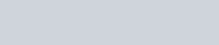Volume of Solutions Exercises

Question 1 (1 point)

A solution is prepared by dissolving 23.1 g of CaC12 (MW = 110.98 g/mol) in 325 mL of water. The density of the resulting solution is 1.05 g/mL. The concentration of CaC12 in this solution is molar.

Answer accurate to three decimal places.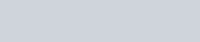Question 2 (1 point)

A solution is prepared by adding 0.8 mol of KCI (MW = 74.55 g/mol) to 879 g of water. The concentration of

KCI is molal.

Answer accurate to three decimal places.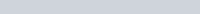The decomposition of N205 proceeds via the reaction:

2 N205 -Y 4 N02 + 02

The reaction is first order and has a rate constant of 4.82 x 10-3 s-1 at 64 cc.

The rate law for the reaction is rate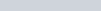o

k

# IN0214 1021

IN-,0512

O k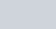[02)

#C)

k

## IN20512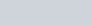1021

O klN20512

o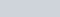Question 4 (1 point)

A first-order reaction has a rate constant of 0.33 min-I . It takesmin for the reactant concentration to decrease from 0.27 M to 0.09 M.

Give answer to two decimal places.

A second-order reaction has a half-life of 10 s when the initial concentration of reactant is 0.95 M. The rate constant for this reaction isM-ls-l.

Give answer accurate to two decimal places.Question 6 (3 points)

For the following reaction, concentration data was collected.

Experiment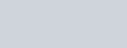Initial Rate

Number

1. 0.273 0.763 2.83
2. 0.273 1.526 2.83
3. 0.819 0.763 25.47

The order of the reaction in A is: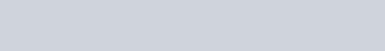Question 8

For the reaction 2 H20 (l)

= [H2]2 [021

## = [H20]2

OIH2121021 IH2012

O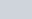[H212 1012 IH2012

2H2 (g) + 02 (g), the equilibrium expression is:

Question 7 (1 point)

The K for the equilibrium below is 7.04 x 10-2 at 480.0 D C.

2 C12 (g) + 2 1-120 (g) +4 HCI (g) + 02 (g)

What is the value of K at this temperature for the following reaction?

2 HCI (g) + 1/2 02 (g) —+ C12 (g) + H20 (g)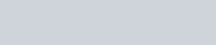Question 9

The pH of 0.010 M trimethylamine is 10.74 at 25 cc. What is the hydroxide ion [-OH] concentration?

Give answer to 5 decimal places (sorry I can’t figure out scientific notation on D2L).Question 10 (1 point)

The pH of a 0.5 M aqueous solution of formic acid, HCH02, at 25.0 oc is 2.0. What is the value of Ka for formic acid?

Give answer to 5 decimal places (sorry I can’t figure out scientific notation on D2L).11

The acid-dissociation constant, Kä, for gallic acid is 5.24 x 10-10. What is the basedissociation constant, Kb, for the gallate ion? Give answer accurate to 6 decimal places.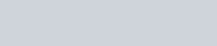Question 12 (1 point)

Calculate the pH of a solution prepared by dissolving 0.27 mol of benzoic acid(C7H502H) and 0.15 mol of sodium benzoate (NaC7H502) in water sufficient to yield 1.00 L Of solution. The Ka Of benzoic acid is 6.50 x 10-5. Give your answer accurate to two decimal places.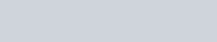Question 13 (1 point)

39 mL of 0.14 M acetic acid solution is titrated with 0.10 M NaOH. What is the pH after 18 mL of NaOH have been added? (Ka for acetic acid = 1.8 x 10-5)

Give your answer accurate to 2 decimal places.

## 15

Given the following information, Calculate delta GOGive your answer in kJ.Ag+(aq) 0.80 v Cr3+(aq) + 3 e – * Cr (s) -0.74 v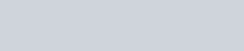Question 14 (1 point)

Consider an unbalanced equation between copper (l) ions and iron to produce copper metal and iron (Il) ions. What is oxidized in this reaction?

Fe (s) Cl.l+ Fe2+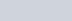(s)C) Fe (s)

C) Cu (s)

O Fe2+

16

Beginning with 85 g of iron-55, calculate how much remains after 7.4 years. The half-life of iron-55 is 2.74 years.

Give your answer accurate to one decimal place.